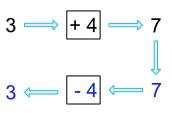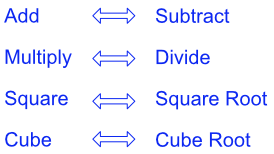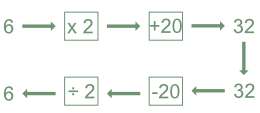Inverse Operations

## Inverse Operations

Most mathematical operations can be reversed: the operation that undoes the original calculation is known as the inverse operation.

A calculation of 3 + 4 gives a result of 7.  The inverse operation starts with the answer (7).  The opposite of adding is subtracting, so subtract 4 from 7 to get back to the original number.Inverse operations work both ways: subtraction is the inverse of addition, and addition is the inverse of subtraction.  Some of the inverse operations that need to be known are:## Example 1

What are the inverse operations for 6 x 2 + 20 = 32?Answer: Subtract 20; then divide by 2, or (32 - 20) ÷ 2 = 6

## Example 2

Two adults and two children went on a holiday.  The total cost of the holiday was £900.  The cost for the adults was £290 each.  How much was the ticket for each child?

2 x adult + 2 x children = £900

2 x adult = 2 x 290 = £580

£900 - £580 = £320 (the inverse of adding the adults tickets to the cost)

£320 ÷ 2 = £160 (the inverse of multiplying by 2 for the two children.